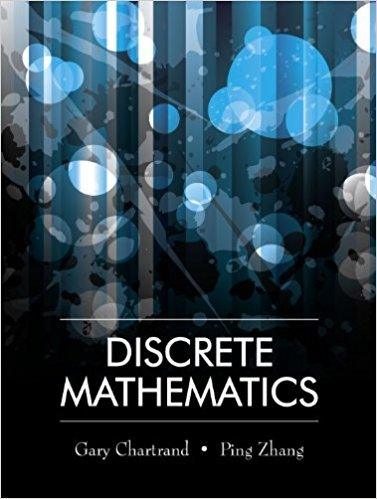×
×

# Solutions for Chapter 8.5: Applications of Permutations and Combinations## Full solutions for Discrete Mathematics | 1st Edition

ISBN: 9781577667308Solutions for Chapter 8.5: Applications of Permutations and Combinations

Solutions for Chapter 8.5
4 5 0 234 Reviews
24
5
##### ISBN: 9781577667308

This expansive textbook survival guide covers the following chapters and their solutions. Since 13 problems in chapter 8.5: Applications of Permutations and Combinations have been answered, more than 11956 students have viewed full step-by-step solutions from this chapter. Chapter 8.5: Applications of Permutations and Combinations includes 13 full step-by-step solutions. This textbook survival guide was created for the textbook: Discrete Mathematics, edition: 1. Discrete Mathematics was written by and is associated to the ISBN: 9781577667308.

Key Math Terms and definitions covered in this textbook
• Big formula for n by n determinants.

Det(A) is a sum of n! terms. For each term: Multiply one entry from each row and column of A: rows in order 1, ... , nand column order given by a permutation P. Each of the n! P 's has a + or - sign.

• Change of basis matrix M.

The old basis vectors v j are combinations L mij Wi of the new basis vectors. The coordinates of CI VI + ... + cnvn = dl wI + ... + dn Wn are related by d = M c. (For n = 2 set VI = mll WI +m21 W2, V2 = m12WI +m22w2.)

• Characteristic equation det(A - AI) = O.

The n roots are the eigenvalues of A.

• Cholesky factorization

A = CTC = (L.J]))(L.J]))T for positive definite A.

• Complex conjugate

z = a - ib for any complex number z = a + ib. Then zz = Iz12.

• Determinant IAI = det(A).

Defined by det I = 1, sign reversal for row exchange, and linearity in each row. Then IAI = 0 when A is singular. Also IABI = IAIIBI and

• Echelon matrix U.

The first nonzero entry (the pivot) in each row comes in a later column than the pivot in the previous row. All zero rows come last.

• Hessenberg matrix H.

Triangular matrix with one extra nonzero adjacent diagonal.

• Inverse matrix A-I.

Square matrix with A-I A = I and AA-l = I. No inverse if det A = 0 and rank(A) < n and Ax = 0 for a nonzero vector x. The inverses of AB and AT are B-1 A-I and (A-I)T. Cofactor formula (A-l)ij = Cji! detA.

• Left nullspace N (AT).

Nullspace of AT = "left nullspace" of A because y T A = OT.

• Nullspace matrix N.

The columns of N are the n - r special solutions to As = O.

• Pascal matrix

Ps = pascal(n) = the symmetric matrix with binomial entries (i1~;2). Ps = PL Pu all contain Pascal's triangle with det = 1 (see Pascal in the index).

• Permutation matrix P.

There are n! orders of 1, ... , n. The n! P 's have the rows of I in those orders. P A puts the rows of A in the same order. P is even or odd (det P = 1 or -1) based on the number of row exchanges to reach I.

• Plane (or hyperplane) in Rn.

Vectors x with aT x = O. Plane is perpendicular to a =1= O.

• Positive definite matrix A.

Symmetric matrix with positive eigenvalues and positive pivots. Definition: x T Ax > 0 unless x = O. Then A = LDLT with diag(D» O.

• Projection matrix P onto subspace S.

Projection p = P b is the closest point to b in S, error e = b - Pb is perpendicularto S. p 2 = P = pT, eigenvalues are 1 or 0, eigenvectors are in S or S...L. If columns of A = basis for S then P = A (AT A) -1 AT.

• Reduced row echelon form R = rref(A).

Pivots = 1; zeros above and below pivots; the r nonzero rows of R give a basis for the row space of A.

• Skew-symmetric matrix K.

The transpose is -K, since Kij = -Kji. Eigenvalues are pure imaginary, eigenvectors are orthogonal, eKt is an orthogonal matrix.

• Symmetric factorizations A = LDLT and A = QAQT.

Signs in A = signs in D.

• Vandermonde matrix V.

V c = b gives coefficients of p(x) = Co + ... + Cn_IXn- 1 with P(Xi) = bi. Vij = (Xi)j-I and det V = product of (Xk - Xi) for k > i.

×